CE433 Embedded Devices Spring 2022
Homework 4 - Data Storage Units
Name: Ryan Jeanes

Email: rejeanes@fortlewis.edu

Homework 4 - Data Storage UnitsFigure 1 - Code for SR latch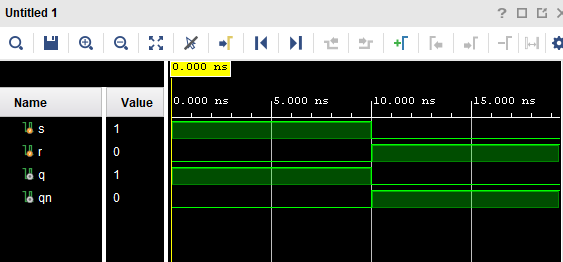Figure 2 - Simulation of SR latchFigure 3 - Code for SR FF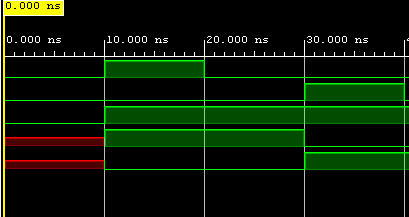Figure 4 - Simulation for SR FF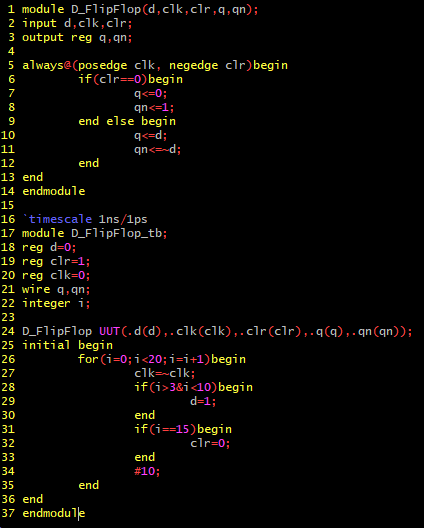Figure 5 - Code for D Flip Flop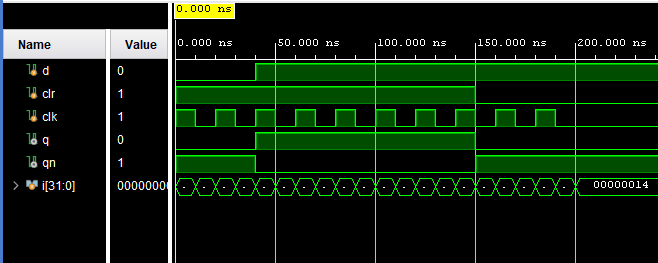Figure 6 - Simulation for D Flip Flop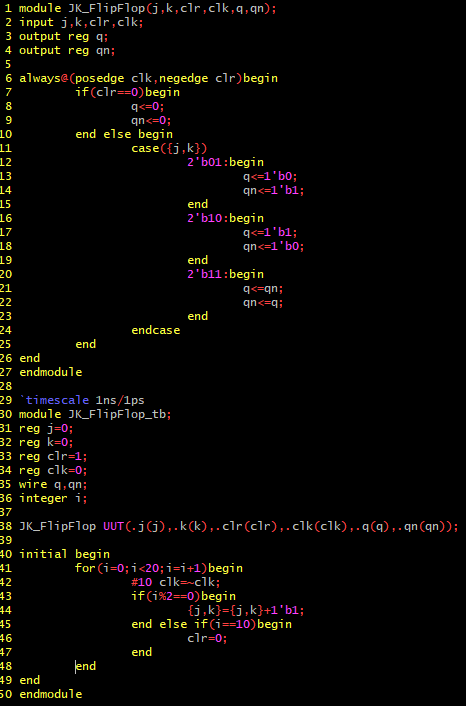Figure 7 - Code for JK Flip Flop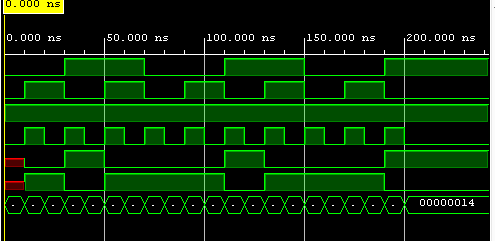Figure 8 - JK Flip Flop simulation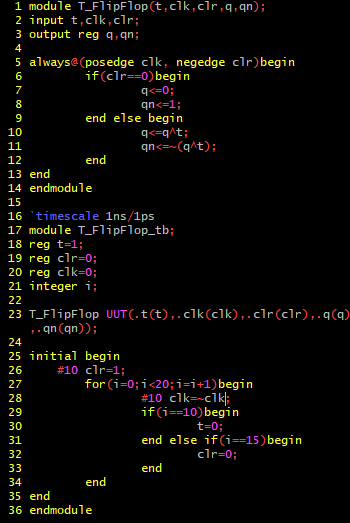Figure 9 - Code for T Flip FlopFigure 10 - Simulation for T Flip Flop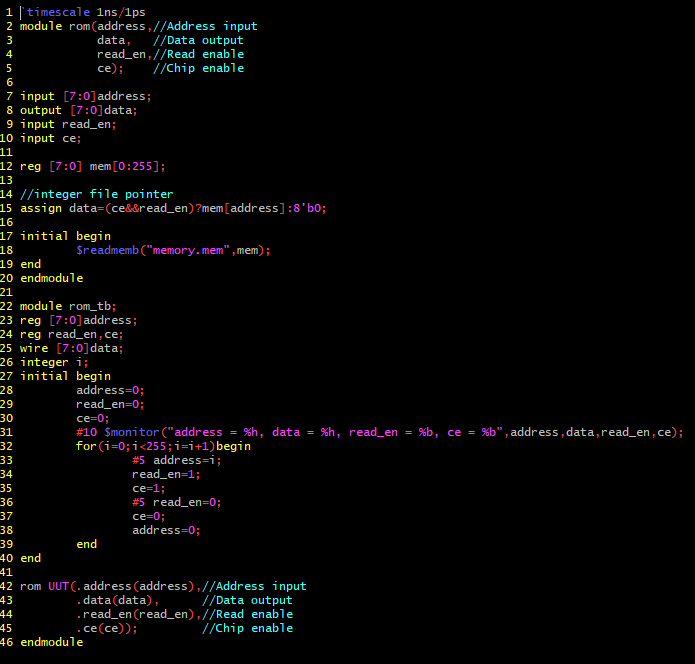Figure 11 -
Code for first ROM example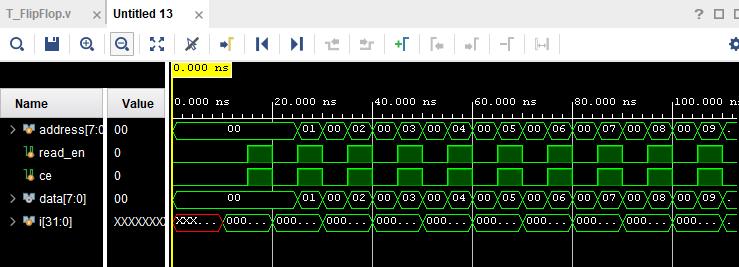Figure 12 - Simulation for first ROM example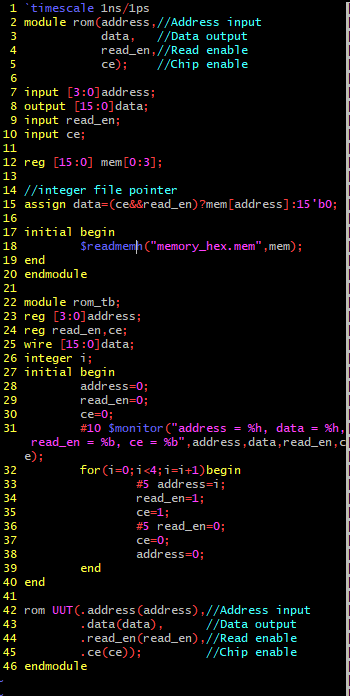Figure 12 - Code for ROM example reading hex values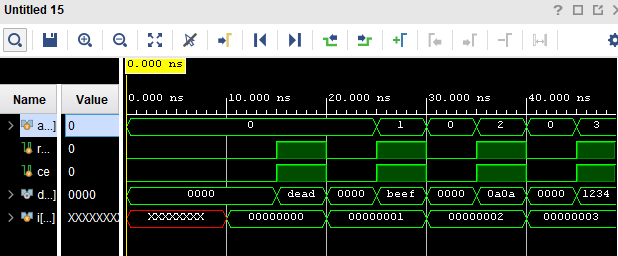Figure 13 - Simulation for hex ROM example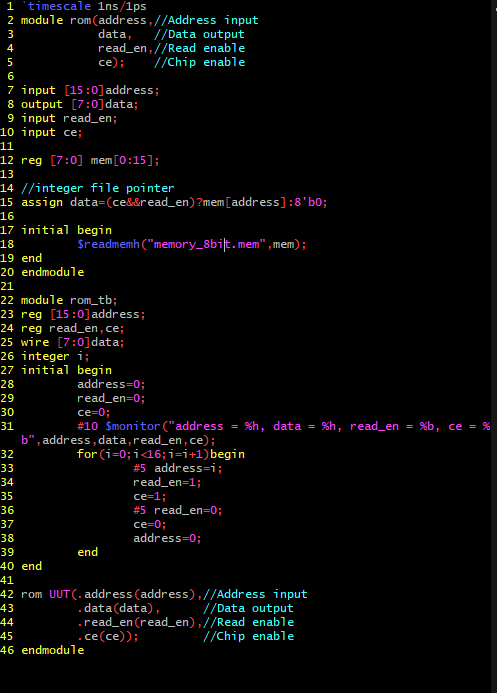Figure 14 - Code for 8bit ROM exampleFigure 15 - Simulation for 8bit ROM example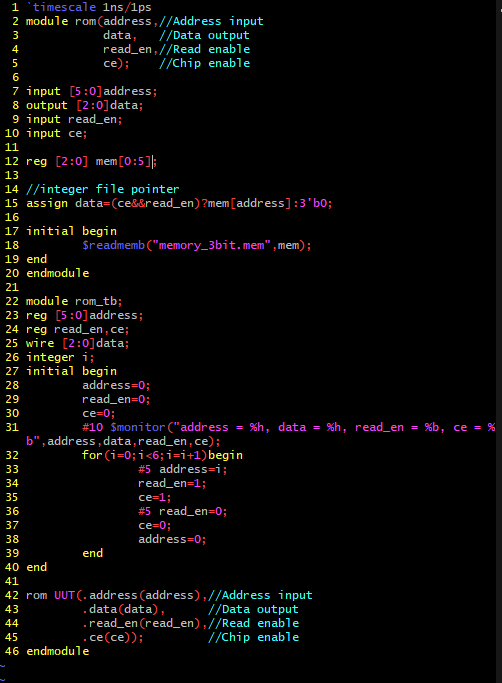Figure 16 - Code for 3bit ROM example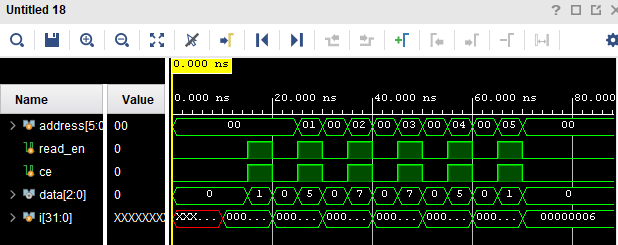Figure 17 - Simulation for 3bit ROM exampleFigure 18 - Code for ROM example using ROM IP Core. Unfortunately, doesn't work.